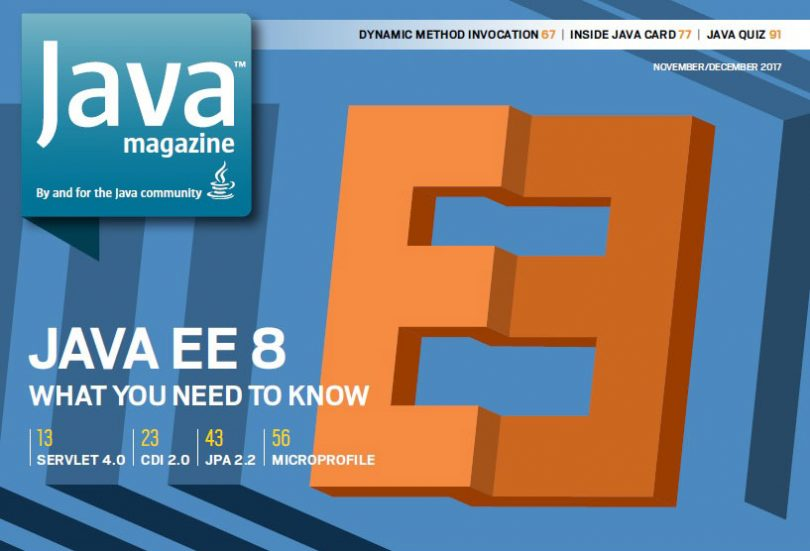News

# November/December 2017 issue of Java Magazine, “Quiz Yourself” (OCA, OCP)Written by

In November/December 2017 issue of Java Magazine was released. There are four interesting questions about OCA (intermediate) and OCP (advanced) Java SE 8 exams:

Question 1 (intermediate). Given this code:

`int a = 012; // line n1`
`int b = 12;`
`int c = Integer.parseInt("012", 10);`

and these statements:

1. `a == b`
2. `b == c`
3. `a == c`
4. `Line n1` causes a compilation error.

Which is true? Choose one.
A. 4 only
B. 1, 2, and 3
C. 1 only
D. 2 only
E. 3 only

Question 2 (intermediate). Given this fragment (shown with line numbers):

`14: long i1 = 1234567890123456789;`
`15: short s1 = 99, s2 = 100, s3 = s1 + s2;`
`16: float pi = 3.14;`
`17: short s = 199;`

Which is true? Choose one.

A. Lines `14` and `16` are correct, but lines `15` and `17` cause compilation to fail.
B. Lines `14` and `15` are correct, but lines `16` and `17` cause compilation to fail.
C. Lines `15` and `17` are correct, but lines `14` and `16` cause compilation to fail.
D. Line `17` is correct, but lines `14`, `15`, and `16` cause compilation to fail.
E. Line `14` is correct, but lines `15`, `16`, and `17` cause compilation to fail.

Question 3 (advanced). Given that the following declaration has been properly initialized to refer to a `List` implementation that contains multiple `String` objects, and that your intention is to remove from the list all strings that start with *:
`List<String> ls;`

Which of the following are true? Choose two.

A. The items can properly be removed by using this code:
`[nbsp count=4]for (String s : ls) {`
`[nbsp count=8]if (s.startsWith("*")) ls.remove(s);`
`[nbsp count=4]}`

B. The items can properly be removed by using this code:
`[nbsp count=4]ls.forEach(s->{if (s.startsWith("*")) ls.remove(s);});`

C. The items can properly be removed by using this code:
`[nbsp count=4]ListIterator<String> lis = ls.listIterator();`
`[nbsp count=4]while (lis.hasNext()) {`
`[nbsp count=8]if (lis.next().startsWith("*")) lis.remove();`
`[nbsp count=4]}`

D. The items can properly be removed by using this code:
`[nbsp count=4]int last = ls.size();`
`[nbsp count=4]for (int idx = 0; idx < last; idx++) {`
`[nbsp count=8]if (ls.get(idx).startsWith("*")) {`
`[nbsp count=12]ls.remove(idx);`
`[nbsp count=8]}`
`[nbsp count=4]}`

E. Given the information available, it’s not possible to guarantee that the removal will succeed.

Question 4 (advanced). Given the following code:

`Optional`
`[nbsp count=4].<String>ofNullable(null)`
`[nbsp count=4].flatMap(x->x != null ? Optional.of("A") : Optional.of("B"))`
`[nbsp count=4].map(String::toLowerCase)`
`[nbsp count=4].ifPresent(System.out::println);`

Which is true? Choose one.

A. The output is `a`.
B. The output is `b`.
C. Replacing the `flatMap` call with `.orElseGet(()->Optional.of("C"))` results in the output `c`.
D. The code runs but produces no output.
E. Replacing the call to `flatMap` with `.map(x->x != null ? "A" : "B")` results in the output `b`.

1.  D
2.  D
3.  C, E
4.  D

These questions are explained detailed by Simon Roberts. You can look at them by clicking the following original link: